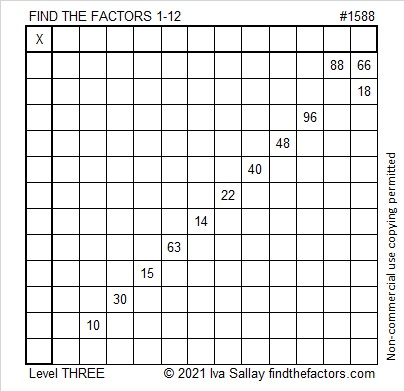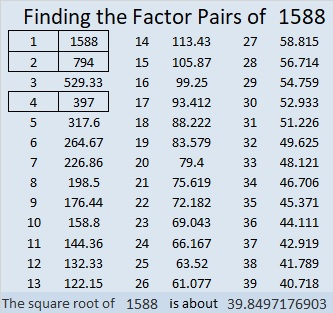# 1588 Cupid’s Arrow

### Today’s Puzzle:

Keeping with our Valentine’s theme, today’s level 3 puzzle looks like Cupid’s Arrow. Start with the clues at the top of the arrow, write in their factors, and work your way down the puzzle, cell by cell, writing in factors as you go. Before long, you will be smitten with this puzzle!### Factors of 1588:

• 1588 is a composite number.
• Prime factorization: 1588 = 2 × 2 × 397, which can be written 1588 = 2² × 397.
• 1588 has at least one exponent greater than 1 in its prime factorization so √1588 can be simplified. Taking the factor pair from the factor pair table below with the largest square number factor, we get √1588 = (√4)(√397) = 2√397.
• The exponents in the prime factorization are 2 and 1. Adding one to each exponent and multiplying we get (2 + 1)(1 + 1) = 3 × 2 = 6. Therefore 1588 has exactly 6 factors.
• The factors of 1588 are outlined with their factor pair partners in the graphic below.### More about the number 1588:

1588 is the difference of two squares:
398² – 396² = 1588.

1588 is also the sum of two squares:
38² + 12² = 1588.

1588 is the hypotenuse of a Pythagorean triple:
912-1300-1588, calculated from 2(38)(12), 38² – 12², 38² + 12².
It is also 4 times (228-325-397).

# 1030 Cupid’s Arrow

Love can often be like a puzzle. When cupid’s arrow hits its mark, at first everything might seem to fall into place, but before long, love starts getting complicated and has to be figured out.

That’s the way this Cupid’s Arrow puzzle is, too. It’s easy to find the logic to start it, but then the logic will be more difficult to see. May you be able to figure out this puzzle as well as the important relationships in your life!Print the puzzles or type the solution in this excel file: 12 factors 1028-1034

What can I tell you about the number 1030?

It’s the sum of two consecutive prime numbers:
509 + 521 = 1030

It’s the hypotenuse of a Pythagorean triple:
618-824-1030 which is (3-4-5) times 206

It’s palindrome 1102011 in BASE 3 because 3⁶ + 3⁵ + 2(3³) + 3¹ + 3⁰ = 1030

• 1030 is a composite number.
• Prime factorization: 1030 = 2 × 5 × 103
• The exponents in the prime factorization are 1, 1, and 1. Adding one to each and multiplying we get (1 + 1)(1 + 1)(1 + 1) = 2 × 2 × 2 = 8. Therefore 1030 has exactly 8 factors.
• Factors of 1030: 1, 2, 5, 10, 103, 206, 515, 1030
• Factor pairs: 1030 = 1 × 1030, 2 × 515, 5 × 206, or 10 × 103
• 1030 has no square factors that allow its square root to be simplified. √1030 ≈ 32.09361